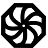# Programming-Idioms# Idiom #24 Assign to string the japanese word ネコ

Declare a new string s and initialize it with the literal value "ネコ" (which means "cat" in japanese)

`let s = "ネコ";`
`const char * s = "ネコ";`
`(def s "ネコ")`
`#include <string>`
`std::string s = u8"ネコ";`
`string s = "ネコ";`
`string s = "ネコ";`
`var s = "ネコ";`
`s = "ネコ"`
`S = unicode:characters_to_binary("ネコ"),`
```  use, intrinsic :: iso_fortran_env
implicit none
integer, parameter :: u = selected_char_kind('ISO_10646')

character(kind=u,len=2) :: cat
cat = u_"ネコ"
```
`s := "ネコ"`
```s = "ネコ"
```
`s = "ネコ";`
`String s = "ネコ";`
`s = 'ネコ'`
`\$s = "ネコ";`
```{\$codepage utf8}
s := "ネコ";```
`use utf8;`
`my \$s = 'ネコ';`
`s = "ネコ"`
`Dim s As String = "ネコ"`
`s = "ネコ"`
`val s = "ネコ"`

#### Idiom created by

programming-idioms.org